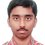Number Theory problem

For how many positive integral values of N is the expression N(N-101) the perfect square of a positive integer ?

I got 2601 as the only value. I want to know if there are other such values ? I know it's a silly question for Brilliant problem solvers. Please help.........Note by Nishant Sharma
6 years, 4 months ago

This discussion board is a place to discuss our Daily Challenges and the math and science related to those challenges. Explanations are more than just a solution — they should explain the steps and thinking strategies that you used to obtain the solution. Comments should further the discussion of math and science.

When posting on Brilliant:

• Use the emojis to react to an explanation, whether you're congratulating a job well done , or just really confused .
• Ask specific questions about the challenge or the steps in somebody's explanation. Well-posed questions can add a lot to the discussion, but posting "I don't understand!" doesn't help anyone.
• Try to contribute something new to the discussion, whether it is an extension, generalization or other idea related to the challenge.
• Stay on topic — we're all here to learn more about math and science, not to hear about your favorite get-rich-quick scheme or current world events.

MarkdownAppears as
*italics* or _italics_ italics
**bold** or __bold__ bold

- bulleted
- list

• bulleted
• list

1. numbered
2. list

1. numbered
2. list
Note: you must add a full line of space before and after lists for them to show up correctly
paragraph 1

paragraph 2

paragraph 1

paragraph 2

> This is a quote
This is a quote
# I indented these lines
# 4 spaces, and now they show
# up as a code block.

print "hello world"
# I indented these lines
# 4 spaces, and now they show
# up as a code block.

print "hello world"
MathAppears as
Remember to wrap math in $$...$$ or $...$ to ensure proper formatting.
2 \times 3 $2 \times 3$
2^{34} $2^{34}$
a_{i-1} $a_{i-1}$
\frac{2}{3} $\frac{2}{3}$
\sqrt{2} $\sqrt{2}$
\sum_{i=1}^3 $\sum_{i=1}^3$
\sin \theta $\sin \theta$
\boxed{123} $\boxed{123}$

Sort by:

Let $x^2=N$ and

$y^2=N-101$ where $x>0,y>0$

Now we can write from our first statement that :

$y^2=x^2-101$

$\Rightarrow x^2-y^2=101$

$(x+y)(x-y)=101$

Therefore it follows that $(x+y)$ and $(x-y)$ are factors of $101$.

Now since $101$ is a prime and $x,y$ are positive integers then the only possible values that $(x+y)$ can take is $101$.

Note that since $x>y$ $,$ $(x-y) \neq 101$.

So the only solution possible is when $x=51$ which gives $y=50$.

Therefore $N=x^2=2601$ is the only solution.

- 6 years, 4 months ago

You need to substantiate your first line. Why must we have $N=x^2$? You are merely given that $N$ is an integer, and not told that it is a square. For example, if we ignore the condition that $N(N-101)$ must be positive, then $N=101$ will be a solution, but that disagrees with your claim that $N$ must be a perfect square.

Staff - 6 years, 4 months ago

If $N=101$ is a solution then $y=0$ but I already stated that $y>0$. So N=101 cannot be a solution.

- 6 years, 4 months ago

it came in isi right

- 6 years, 4 months ago

yea,you too gave isi,how many could you answer in part i & II...?

- 6 years, 4 months ago

Yeah it was an ISI problem. Well I attempted 29/30 in part I and solved 4 in part II. How about you both ?

- 6 years, 4 months ago

i am 13 so i cannot participate but i saw the question and tried to solve it . i got about 28 in part 1 and 7 in part 2

- 6 years, 4 months ago

Are you sure or just kidding(your age) ? Anyway, where from did you get the questions ?

- 6 years, 4 months ago

y kiddin and q from http://cheentaganitkendra.blogspot.in/2013/05/isi-2013-bmath-bstat-subjective-paper.html

- 6 years, 4 months ago

You are missing another obvious (positive integral) solution. (This statement is false. See edit below.)

Hint: Greatest Common Divisor.

Edit: I missed out that $N (N-101)$ has to be the square of a positive integer. I was thinking that $N=101$ works.

Staff - 6 years, 4 months ago

I don't think that any other solution exists, Calvin Sir. Both $N$ and $N(N-101)$ are to be positive.

- 6 years, 4 months ago

I misread, didn't see "positive" in the latter instance. Thanks for correcting.

Staff - 6 years, 4 months ago

Me too. That was why I posted this since I had done few problems only. BTW did you also write ISI 2013 ?

- 6 years, 4 months ago

yes

- 6 years, 4 months ago

Seriously could not follow you. Please extrapolate a bit.

- 6 years, 4 months ago